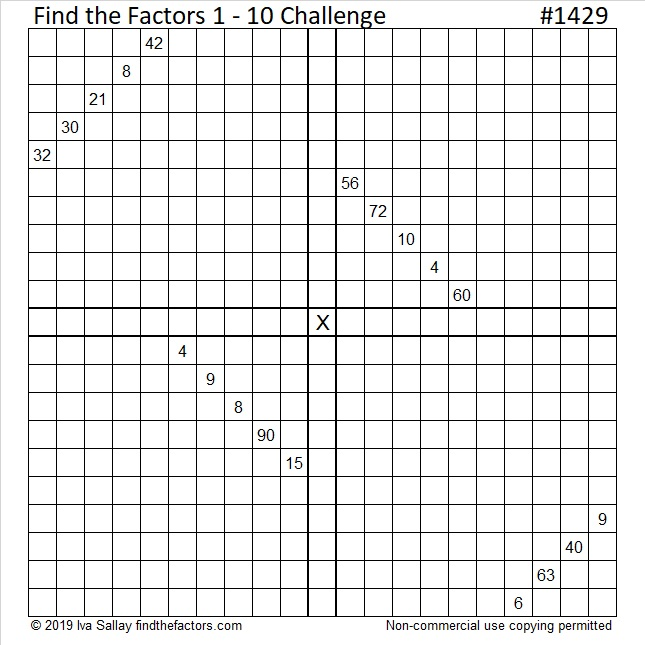# 1429 Find the Factors Challenge

I wanted today’s puzzle to look like a big candy bar, but I don’t think I succeeded. I hope you will still think it is the best treat you got today! Good luck!Print the puzzles or type the solution in this excel file: 12 Factors 1419-1429

Now I’ll tell you some facts about the number 1429:

• 1429 is a prime number.
• Prime factorization: 1429 is prime.
• 1429 has no exponents greater than 1 in its prime factorization, so √1429 cannot be simplified.
• The exponent in the prime factorization is 1. Adding one to that exponent we get (1 + 1) = 2. Therefore 1429 has exactly 2 factors.
• The factors of 1429 are outlined with their factor pair partners in the graphic below.

How do we know that 1429 is a prime number? If 1429 were not a prime number, then it would be divisible by at least one prime number less than or equal to √1429. Since 1429 cannot be divided evenly by 2, 3, 5, 7, 11, 13, 17, 19, 23, 29, 31, or 37, we know that 1429 is a prime number.OEIS.org informs us that 1429² = 2,042,041. That’s the smallest perfect square whose first three digits are repeated in order by the next three digits.

1429 is the sum of two squares:
30² + 23² = 1429

1429 is the hypotenuse of a primitive Pythagorean triple:
371-1380-1429 calculated from 30² – 23², 2(30)(23), 30² + 23²

Here’s another way we know that 1429 is a prime number: Since its last two digits divided by 4 leave a remainder of 1, and 30² + 23² = 1429 with 30 and 23 having no common prime factors, 1429 will be prime unless it is divisible by a prime number Pythagorean triple hypotenuse less than or equal to √1429. Since 1429 is not divisible by 5, 13, 17, 29, or 37, we know that 1429 is a prime number.

This site uses Akismet to reduce spam. Learn how your comment data is processed.